# Case Study: Synthetic Data

#### 2022-09-23

library(EcoEnsemble)
library(mgcv)
#>
#> Attaching package: 'nlme'
#> The following object is masked from 'package:dplyr':
#>
#>     collapse
#> This is mgcv 1.8-42. For overview type 'help("mgcv-package")'.
library(ggplot2)
library(cowplot)
set.seed(1234)

## Introduction

In this document, we generate synthetic data for simulator outputs and observations, then fit the ensemble model using these data. Finally, we demonstrate the consistency of the ensemble framework and explore uncertainty levels for different numbers of simulators. We do this for an ensemble model of 4 simulators, each describing 3 variables of interest.

## Generating the data

We generate the synthetic data by sampling from an informative prior distribution of the ensemble model. We choose priors with very strong correlations of individual long-term discrepancies ($$\mathrm{Beta}(5,1)$$ via the method of concordance) and high autocorrelations of the AR processes on individual and shared short-term discrepancies ($$\frac{r_i + 1}{2}\sim \mathrm{Beta}(10,2)$$). These are different to the vague priors that will later be used to fit the model. We can configure this prior using the EnsemblePrior() constructor function.

d <- 3 # The number of variables.

priors <- EnsemblePrior(d,
ind_st_params = IndSTPrior(AR_params=c(10,2)),
ind_lt_params = IndLTPrior("beta",
cor_params = list(matrix(5, d, d),
matrix(1, d, d))),
sha_st_params = ShaSTPrior(AR_params=c(10,2)),
)

From this configuration, sampling the ensemble model parameters is done using the prior_ensemble_model() function. In this case we sample the prior of an ensemble model with $$M = 4$$ simulators.

M <- 4 # The number of models we are considering.
prior_density <- prior_ensemble_model(priors, M = M)
ex.fit <- rstan::extract(prior_density$samples) # Extracted samples Of these samples, the data we choose to make up our synthetic data is the single sample which maximizes the likelihood. Recall that for EcoEnsemble, the truth and the discrepancy terms follow a dynamic linear model with $\begin{equation} \mathbf\theta^{(t)}=(\mathbf{y}^{(t)'},\mathbf\eta^{(t)'},\mathbf{z}_1^{(t)'},\ldots,\mathbf{z}_m^{(t)'})', \end{equation}$ so that $\begin{equation} \mathbf\theta^{(t)}|\mathbf\theta^{(t-1)}\sim{}N(A\mathbf\theta^{(t)},\Lambda), \end{equation}$ with $\begin{equation} A= \begin{pmatrix} I_d & 0 & 0 & 0 & \ldots & 0\\ 0 & R_\eta & 0 & 0 & \ldots & 0\\ 0 & 0 & R_1 & 0 & \ldots & 0\\ 0 & 0 & 0 & R_2 & \ldots & 0\\ \vdots & \vdots & \vdots & \vdots & \ddots & \vdots \\ 0 & 0 & 0 & 0 & \ldots & R_m \end{pmatrix}, \end{equation}$ with $$I_d$$ being a $$d$$ dimensional indicator matrix, and $\begin{equation} \Lambda= \begin{pmatrix} \Lambda_y & 0 & 0 & 0 & \ldots & 0\\ 0 & \Lambda_\eta & 0 & 0 & \ldots & 0\\ 0 & 0 & \Lambda_1 & 0 & \ldots & 0\\ 0 & 0 & 0 & \Lambda_2 & \ldots & 0\\ \vdots & \vdots & \vdots & \vdots & \ddots & \vdots \\ 0 & 0 & 0 & 0 & \ldots & \Lambda_m \end{pmatrix}. \end{equation}$ See the EcoEnsemble vignette for further details. The samples from the prior distribution provide us with $$A$$ and $$\Lambda$$ values. To get from these ensemble model parameters to simulated model outputs requires using the above transformations. Values of the truth and discrepancy terms are generated using these values and sums of discrepancy terms to give the “best guesses” of simulator outputs. Time <- 50 true_par <-which.max(ex.fit$lp__)

latent <- matrix(NA, Time, (M+2)*d)

#Priors on initial values
latent[1, 1:d] <- rnorm(d, 0, 1)
latent[1, -(1:d)] <- t(chol(ex.fit$SIGMA_init[true_par,-(1:d) ,-(1:d)])) %*% rnorm((M+1)*d, 0, 1) #Find all the latent values of the dynamic linear model SIGMA <- ex.fit$SIGMA[true_par,,]
SIGMA_chol <- t(chol(SIGMA))
for (t in 2:Time) {
latent[t,] <- ex.fit$AR_params[true_par,] * latent[t-1,] + SIGMA_chol %*% rnorm((M+2)*d, 0, 1) } #The truth is simply the first d values truth_latent <- latent[,1:d] #The best guesses are sums of the truth and discrepancies simulator_best_guesses <- array(NA,dim=c(Time,d,M)) for(i in 1:M){ simulator_best_guesses[,,i] <- t( t(latent[,1:d] + latent[,(d+1):(2*d)] + latent[,(1+i) * d + (1:d)]) + ex.fit$ind_lt[true_par,i,] + ex.fit$sha_lt[true_par,] ) } We can see how the truth relates to simulator best guesses by plotting these outputs, for example for the first variable. plot(truth_latent[,1], ylim=c(-2.5, 3), pch = 19) lines(simulator_best_guesses[,1,1], col = 2) lines(simulator_best_guesses[,1,2], col = 3) lines(simulator_best_guesses[,1,3], col = 4) lines(simulator_best_guesses[,1,4], col = 5)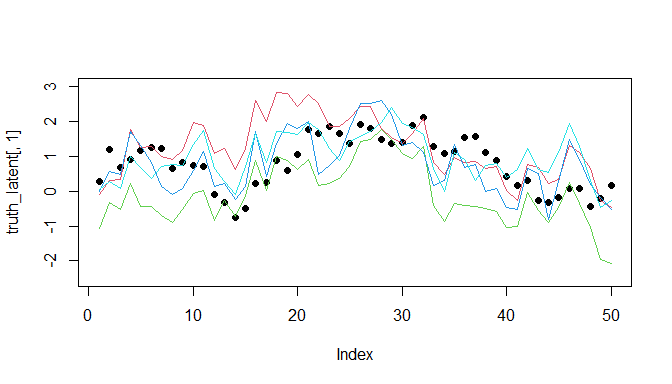In EcoEnsemble, we do not assume that we have access to the truth, nor the model best guesses. Instead, EcoEnsemble assumes that model outputs are noisy representations of the best guesses, and that observations are noisy representations of the truth. In order to produce the final synthetic dataset, we add noise to the simulated truth and best guesses. For simulators, this noise represents parameter uncertainty. We use gams from the mgcv package to achieve this, and we overparametrise the model (by setting $$k$$ - the dimension of the basis used to represent the smooth term - to be something very large) to ensure that the outputs are sufficiently smooth. In addition, we assume that we only have access to the first 40 years of observations. Times_obs <- round(Time * 0.8) obs <- matrix(NA,Times_obs,d) for(i in 1:d){ g1 <- gam(y~s(x,k = 15),data=data.frame(x=1:Times_obs,y = truth_latent[1:Times_obs,i])) obs[,i] <- predict(g1) } obs.cov <- cov(obs - truth_latent[1:Times_obs,]) model.cov <- array(0,dim=c(M,d,d)) models_output <- array(NA,dim=c(M,Time,d)) for (j in 1:M){ for(i in 1:d){ g1 <- gam(y~s(x, k = 15),data=data.frame(x=1:Time,y=simulator_best_guesses[,i,j])) models_output[j,,i] <- predict(g1) } model.cov[j,,] <- cov(models_output[j,,] - simulator_best_guesses[,,j]) } We can see how this data compares to the best guesses by again plotting the first variable of interest. #Observations and model outputs plot(obs[,1], ylim=c(-2.5, 3), xlim = c(1,Time), pch = 19) lines(models_output[1,,1], col=2, lwd = 2) lines(models_output[2,,1], col=3, lwd = 2) lines(models_output[3,,1], col=4, lwd = 2) lines(models_output[4,,1], col=5, lwd = 2)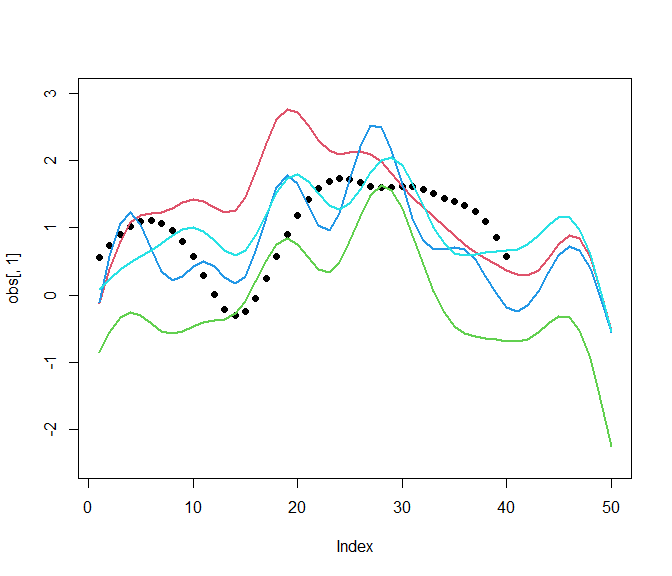In the above graph, dashed lines / empty points represent the best guesses / truth from the ensemble model, while solid lines / filled point represent the simulator outputs / observations. ## Fitting to Synthetic Data We now need to convert the observations and simulator outputs into the required form for EcoEnsemble. This requires that input columns and rows are named consistently with years and species so that data from different models can be matched to each other, and missing data accounted for. We create data frames for each simulator and assign names. #Create the data frames that we'll use for EcoEnsemble val_obs <- data.frame(obs); cov_obs <- obs.cov val_model_1 <- data.frame(models_output[1,,]); cov_model_1 <- model.cov[1,,] val_model_2 <- data.frame(models_output[2,,]); cov_model_2 <- model.cov[2,,] val_model_3 <- data.frame(models_output[3,,]); cov_model_3 <- model.cov[3,,] val_model_4 <- data.frame(models_output[4,,]); cov_model_4 <- model.cov[4,,] #Set the dimnames to ensure EcoEnsemble can identify the information. SPECIES_NAMES <- c("Species 1", "Species 2", "Species 3") dimnames(val_obs) <- list(paste(1:Times_obs), SPECIES_NAMES) dimnames(val_model_1) <- list(paste(1:Time), SPECIES_NAMES) dimnames(val_model_2) <- list(paste(1:Time), SPECIES_NAMES) dimnames(val_model_3) <- list(paste(1:Time), SPECIES_NAMES) dimnames(val_model_4) <- list(paste(1:Time), SPECIES_NAMES) dimnames(cov_obs) <- list(SPECIES_NAMES, SPECIES_NAMES) dimnames(cov_model_1) <- list(SPECIES_NAMES, SPECIES_NAMES) dimnames(cov_model_2) <- list(SPECIES_NAMES, SPECIES_NAMES) dimnames(cov_model_3) <- list(SPECIES_NAMES, SPECIES_NAMES) dimnames(cov_model_4) <- list(SPECIES_NAMES, SPECIES_NAMES) Now we can fit the ensemble model, this time using vague default priors. fit <- fit_ensemble_model(observations = list(val_obs, cov_obs), simulators = list(list(val_model_1, cov_model_1, "Model 1"), list(val_model_2, cov_model_2, "Model 2"), list(val_model_3, cov_model_3, "Model 3"), list(val_model_4, cov_model_4, "Model 4")), priors = EnsemblePrior(d), control = list(adapt_delta = 0.9)) samples <- generate_sample(fit) ## Results We can compare the truth as predicted by the ensemble model with the original truth value. var_index <- 1 df <- data.frame("Year" = paste(1:Time), "Ensemble" = apply(samples@mle[, var_index, ], 1, median), "Lower" = apply(samples@samples[, var_index, ], 1, quantile, 0.1), "Upper" = apply(samples@samples[, var_index, ], 1, quantile, 0.9), "Actual" = truth_latent[,var_index]) df$Year <- as.numeric(df$Year) df <- reshape2::melt(df, id.vars=c("Year", "Lower", "Upper"), variable.name="Simulator") # We only want uncertainty bands for the Ensemble values, else we set zero width bands df[df$Simulator == "Actual", c("Lower", "Upper")] <- df[df\$Simulator != "Actual", "value"]

ggplot(df, aes(x=Year, y=value)) +
geom_line(aes(group=Simulator,colour=Simulator)) +
geom_ribbon(aes(ymin=Lower, ymax =Upper, fill = Simulator), alpha=0.2)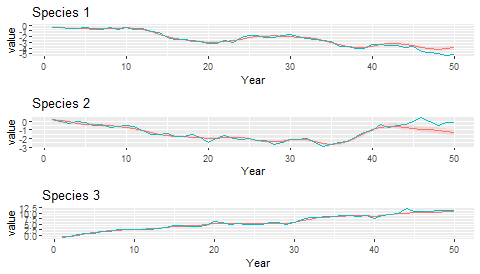Here we see that the ensemble model is able to effectively capture the true value.

## Effect of number of simulators

Unlike other model averaging methods, the ensemble framework allows for uncertainty to decrease as the number of models increases. We can observe this by refitting the ensemble on a smaller number of simulators. We refit the ensemble with $$M = 1, 2, 3$$.

fit_M1 <- fit_ensemble_model(observations = list(val_obs, cov_obs),
simulators = list(list(val_model_1, cov_model_1, "Model 1")),
priors = EnsemblePrior(d),
samples_M1 <- generate_sample(fit_M1)

fit_M2 <- fit_ensemble_model(observations = list(val_obs, cov_obs),
simulators = list(list(val_model_1, cov_model_1, "Model 1"),
list(val_model_2, cov_model_2, "Model 2")),
priors = EnsemblePrior(d),
samples_M2 <- generate_sample(fit_M2)

fit_M3 <- fit_ensemble_model(observations = list(val_obs, cov_obs),
simulators = list(list(val_model_1, cov_model_1, "Model 1"),
list(val_model_2, cov_model_2, "Model 2"),
list(val_model_3, cov_model_3, "Model 3")),
priors = EnsemblePrior(d),
samples_M3 <- generate_sample(fit_M3)

plot_grid(
plot(samples_M1, variable=1) + ggtitle("1 Model")  + theme(legend.position = "none"),
plot(samples_M2, variable=1) + ggtitle("2 Models") + theme(legend.position = "none"),
plot(samples_M3, variable=1) + ggtitle("3 Models") + theme(legend.position = "none"),
plot(samples   , variable=1) + ggtitle("4 Models") + theme(legend.position = "none"))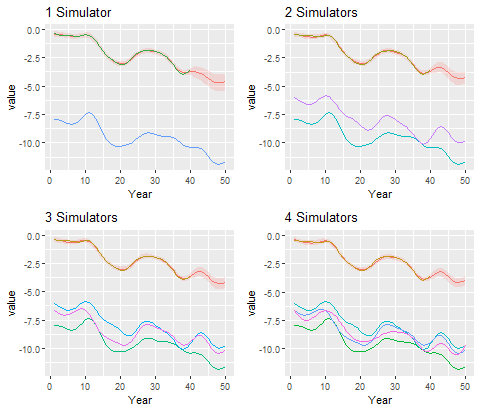To quantify this effect, we plot how the variance of predictions beyond the range of observation data changes over time.

def.par <- par(no.readonly=TRUE) #old pars
par(mfrow = c(d, 1))
legend_ys <- c(0.4, 0.18, 0.12)
for(i in 1:d){
plot.ts(apply(samples_M1@samples[40:50,i,],1, var),
main = paste0("Species ", i), ylab="Variance" )
lines(apply(samples_M2@samples[40:50,i,],1, var), col = 2)
lines(apply(samples_M3@samples[40:50,i,],1, var), col = 3)
lines(apply(samples@samples[40:50,i,],1, var), col = 4)
legend(1, legend_ys[i], legend = c("1 Model", "2 Models", "3 Models", "4 Models"),
col = 1:4, lty = 1)
}
par(def.par)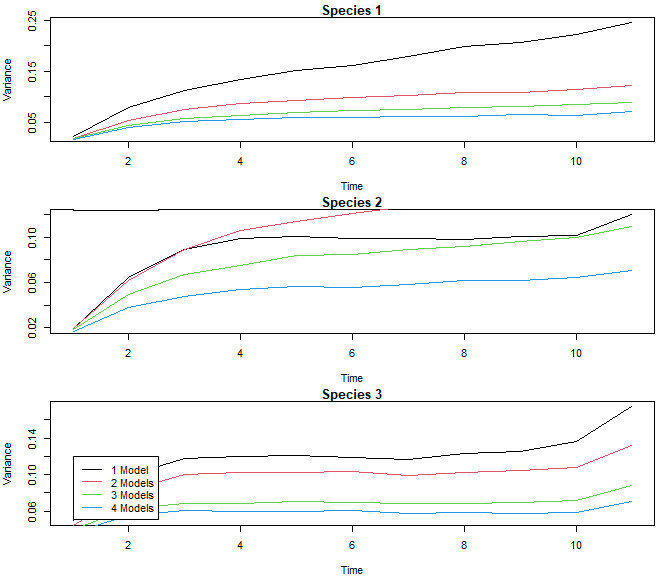As shown in the graphs, there is a marked reduction in the variance of predictions when more simulators are used in the ensemble.